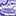# New to Qlik Sense

If you’re new to Qlik Sense, start with this Discussion Board and get up-to-speed quickly.

Announcements
Our next Qlik Insider session will cover new key capabilities. Join us August 11th REGISTER TODAY
cancel
Showing results for
Did you mean:Contributor

## percentage stacked bar chart help

I want to make a percentage stacked bar chart with sales data per territory per month but only for the last year. The last year of data was in 2014. This is where I am currently at.The expression I used in the measure was:

Sum({\$<Year={\$(=max(Year)-1)}>}[LineTotal])

Line total is the order total for a sale.

I need the current component contributed by each region to be expressed as a percentage of its total stack, with the restriction of data only from the most recent year of sales.

1 Solution

Accepted SolutionsMVP

May be this

Sum({\$<Year={\$(=max(Year)-1)}>}[LineTotal])/Sum(TOTAL <DateID.Month> {\$<Year={\$(=max(Year)-1)}>}[LineTotal])

2 RepliesMVP

May be this

Sum({\$<Year={\$(=max(Year)-1)}>}[LineTotal])/Sum(TOTAL <DateID.Month> {\$<Year={\$(=max(Year)-1)}>}[LineTotal])Contributor
Author

You are a legend. Thank you so much. It worked perfectly.Tags
Community Browser